# Calculate Years Between Dates In Ms Excel

If you are an avid Ms Excel user, then you might have come across a task in which you needed to calculate the years between the dates; you might take it easy and do this task manually, which is also feasible but only for calculating the years between 3 to 4 dates. But when it comes to hundreds of dates, it becomes a cumbersome and time taking task to calculate the years between them manually.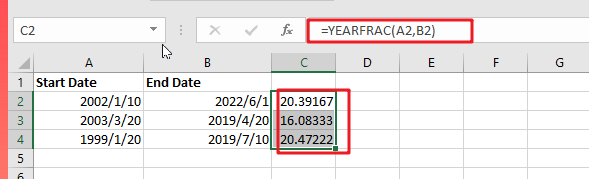But don’t worry since this post will teach you the simplest technique to calculate years between dates in Microsoft Excel. All you have to do is thoroughly read the article.

So without any further ado, let’s dive into it

## General Formula

`=YEARFRAC(start_date,end_date)`

### Explanations Of Syntax:

You must understand the syntax used in this formula in order to apply it to complete your task.

• Parenthesis (): The primary function of this symbol is to group the elements.
• The Comma Symbol (,): This acts as a separator.
• YEARFRAC: It computes the year’s percentage represented by the number of entire days between two dates (start date and end date).

## Summary

The YEARFRAC function, which returns a decimal value indicating the fraction of a year between two dates, may be used to compute the number of years between two dates. The formula in D6 in the example is:

`=YEARFRAC(A2, B2)`

### Explanation

YEARFRAC provides a decimal value that represents the fractional years between two dates. As an example:

`=YEARFRAC("January 1, 2020","January 1, 2022") / yields 2`

Here are a few instances of YEARFRAC’s calculated results:

 Start Date End Date YEARFRAC result 2002/1/10 2022/6/1 20.39167 2003/3/20 2019/4/20 16.08333 1999/1/20 2019/7/10 20.47222

The formula in C2 in the example is: `=YEARFRAC(A2, B2)` / returns 20 Rounding outcome

You can round the number after you obtain the decimal value. For example, you might use the ROUND function to round to the nearest whole number:

`=ROUND(YEARFRAC(A2,B2),0)`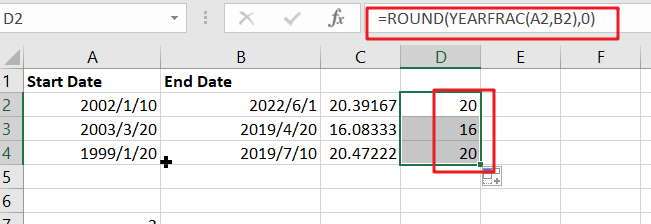Only Whole Years

You may also wish to maintain only the integer component of the result, with no fractional values, to ensure that you only count entire years. In such instance, merely use the INT function to enclose YEARFRAC:

`=INT(YEARFRAC(A2,B2))`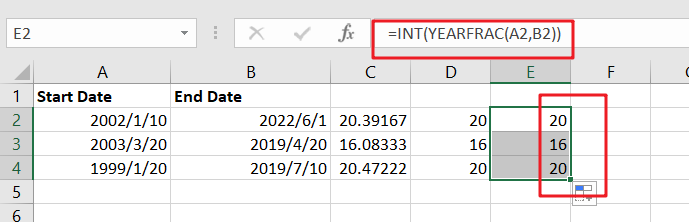Example #2

Assuming you already have the data established in the excel sheets, you do not need to repeat the first step. For those doing it for the very first time, open Excel on your laptop, create a new or empty excel sheet, and record your chosen date values as shown below.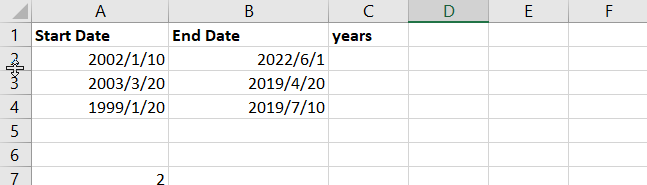We’ll use the YEARFRAC function to calculate the years between the dates on the excel mentioned above. This is the function that computes the number of years between two dates. The YEARFRAC function has the ability to compute in decimal; for example, it can display 1.5 years to imply one and a half years.

Years are where we anticipate recording our outcomes in the column with the title. To compute the years between the dates in cells A2 and B2,

Enter the formula =YEARFRAC (A2, B2) in the formula bar.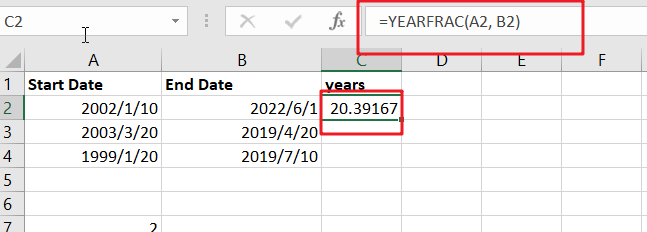Apply the same procedure to the remaining cells, or hold and drag the cursor to compute the remaining years automatically.

Enter this formula in cell D2 and drag the fill handle to get the additional computations.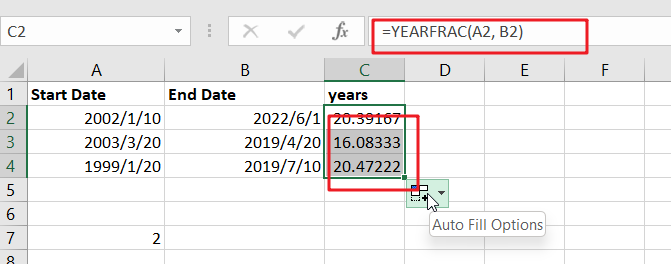To round the decimal or fractional value to the closest whole number, use the ROUND function, such as =ROUND(YEARFRAC(A2, B2),0)the above example shows how to round a fractional value to the nearest whole number.

If we want to return the integer component of the year’s value and not the fractional part, wrap the YEARFRAC function inside the INT function, as in =INT(YEARFRAC(B2, C2)). Figure 4: Obtaining the Year Value Absent Fractional ValueNote: There is an optional third input to the YEARFRAC function that determines how days are tallied when computing fractional years. The default approach is to calculate days between two dates using a 360-day year, with each month having 30 days.

### Related Functions

• Excel INT function
The Excel INT function returns the integer portion of a given number. And it will rounds a given number down to the nearest integer.The syntax of the INT function is as below:= INT (number)…
• Excel Round function
The Excel INT function rounds a number to a specified number of digits. You can use the ROUND function to round to the left or right of the decimal point in Excel.The syntax of the ROUND function is as below:=ROUND (number, num_digits)…